# RD Sharma Solutions for Class 8 Maths Chapter 17 Understanding Shapes- III (Special Types of Quadrilaterals) Exercise 17.3

In Exercise 17.3 of RD Sharma, we mainly study the properties of a rectangle, properties of a square and properties of a trapezium. Exercise wise solutions are formulated by our expert team at BYJU’S in order to analyze the topics in a more effective way. Illustrative examples are also provided to help students comprehend the concepts even better. By referring to RD Sharma Solutions students are advised to practice the solutions regularly to come out with flying colours in their exams. Students can also download the Pdf easily from the links given below.

## Download the Pdf of RD Sharma Solutions for Class 8 Maths Exercise 17.3 Chapter 17 Understanding Shapes- III (Special Types of Quadrilaterals)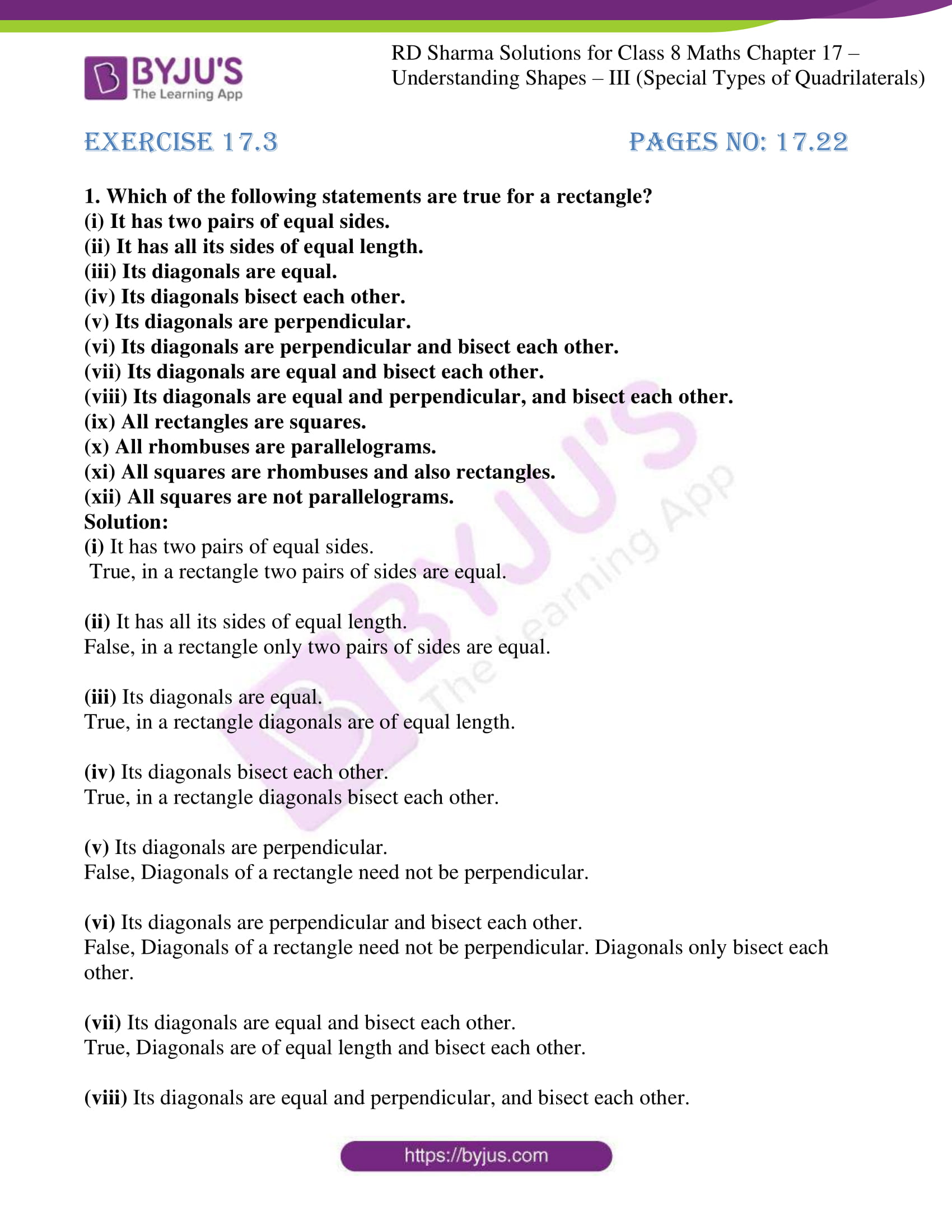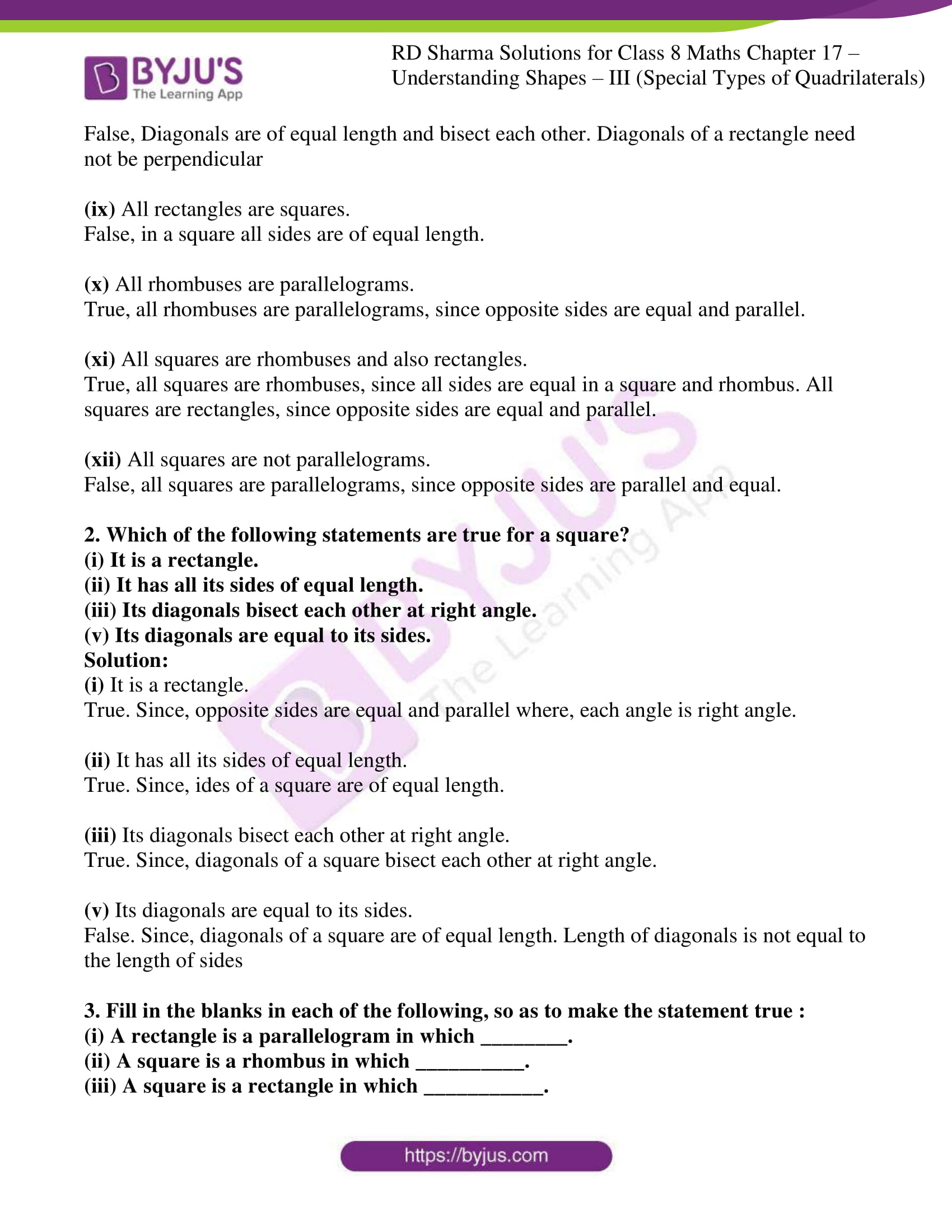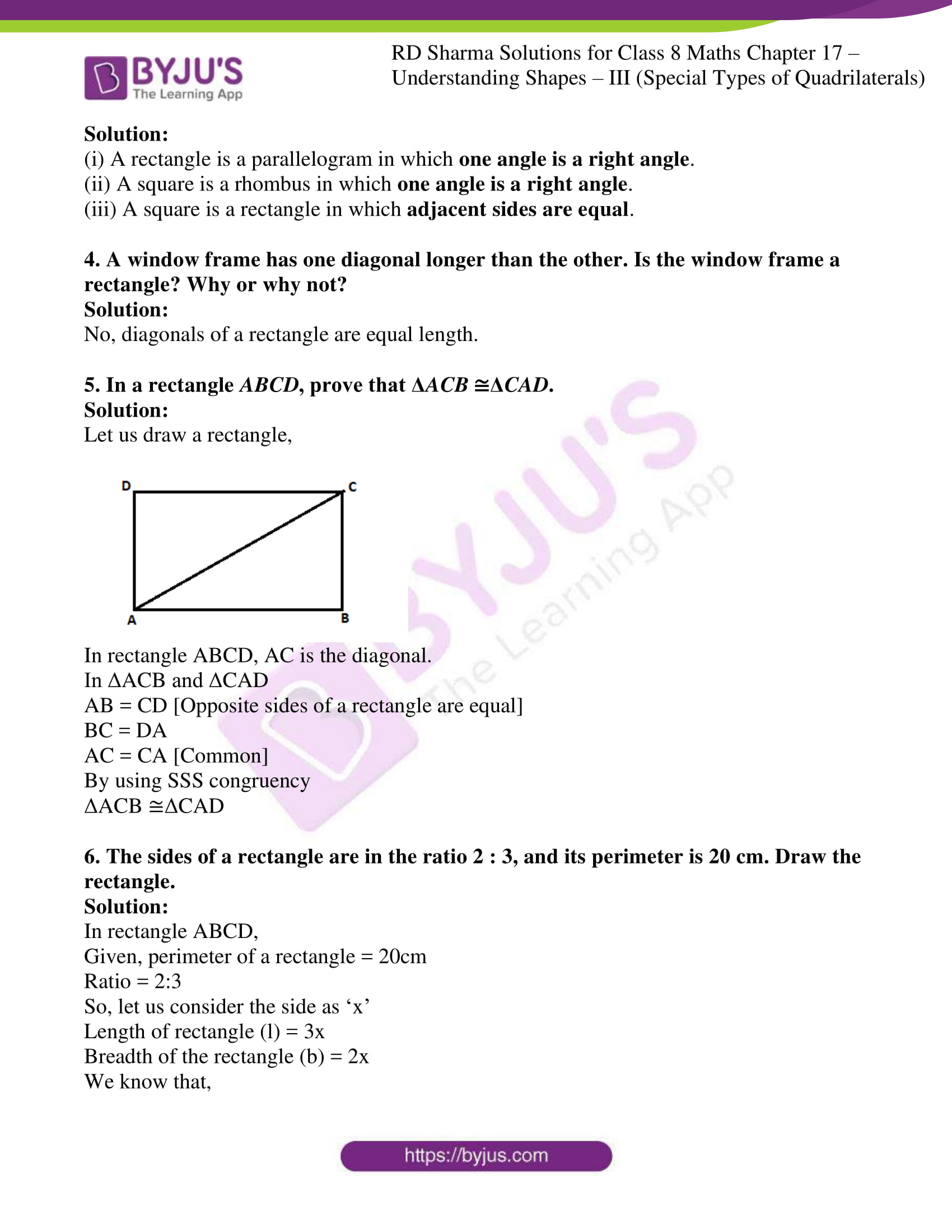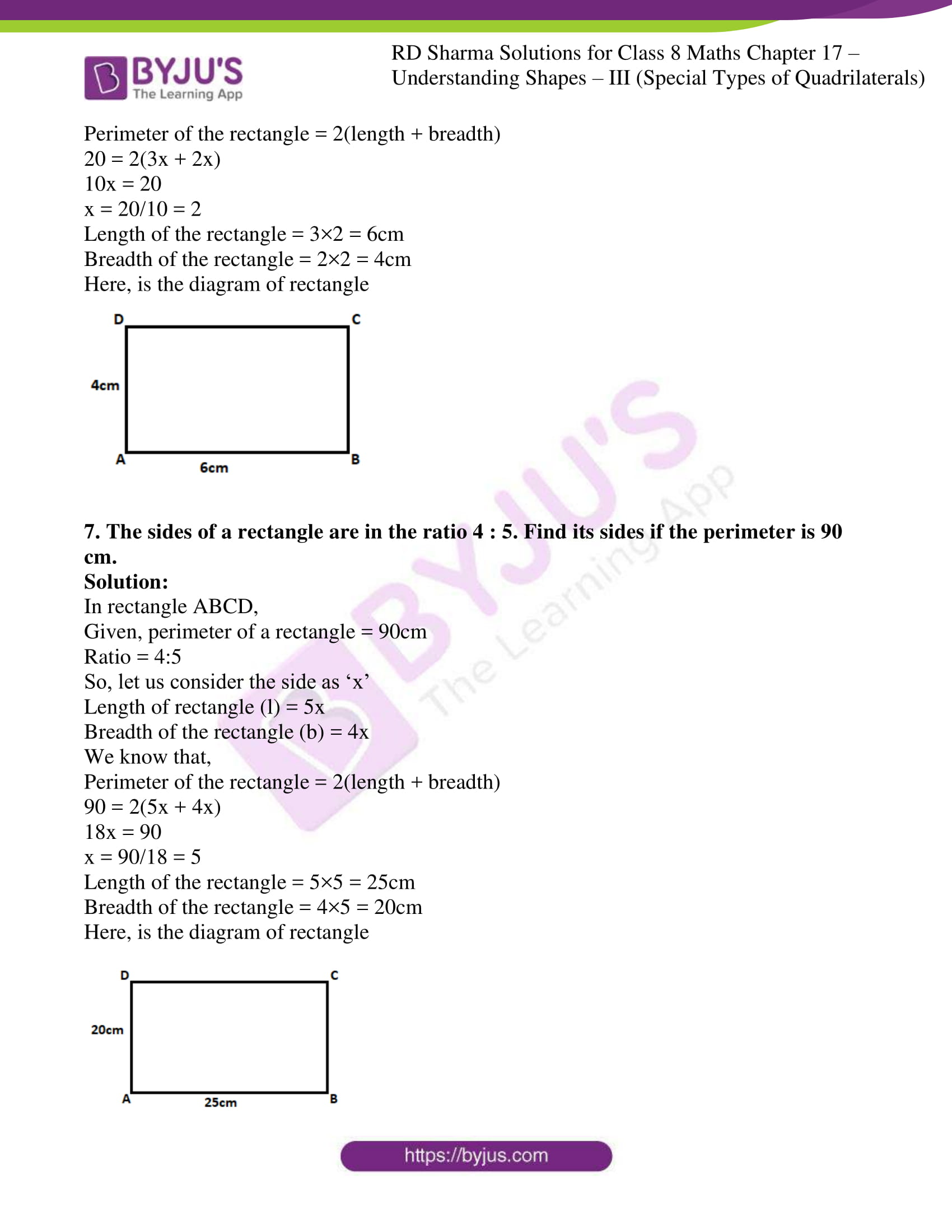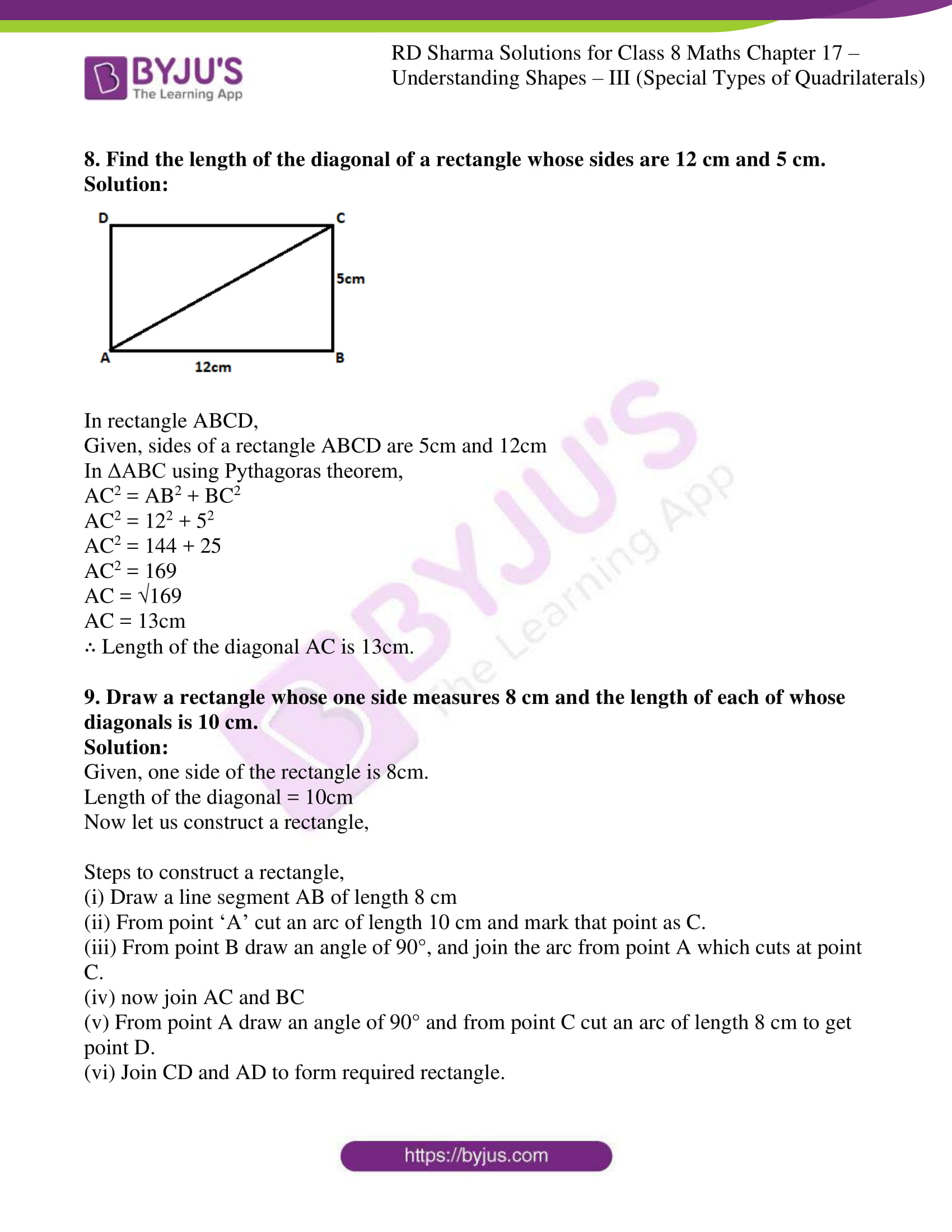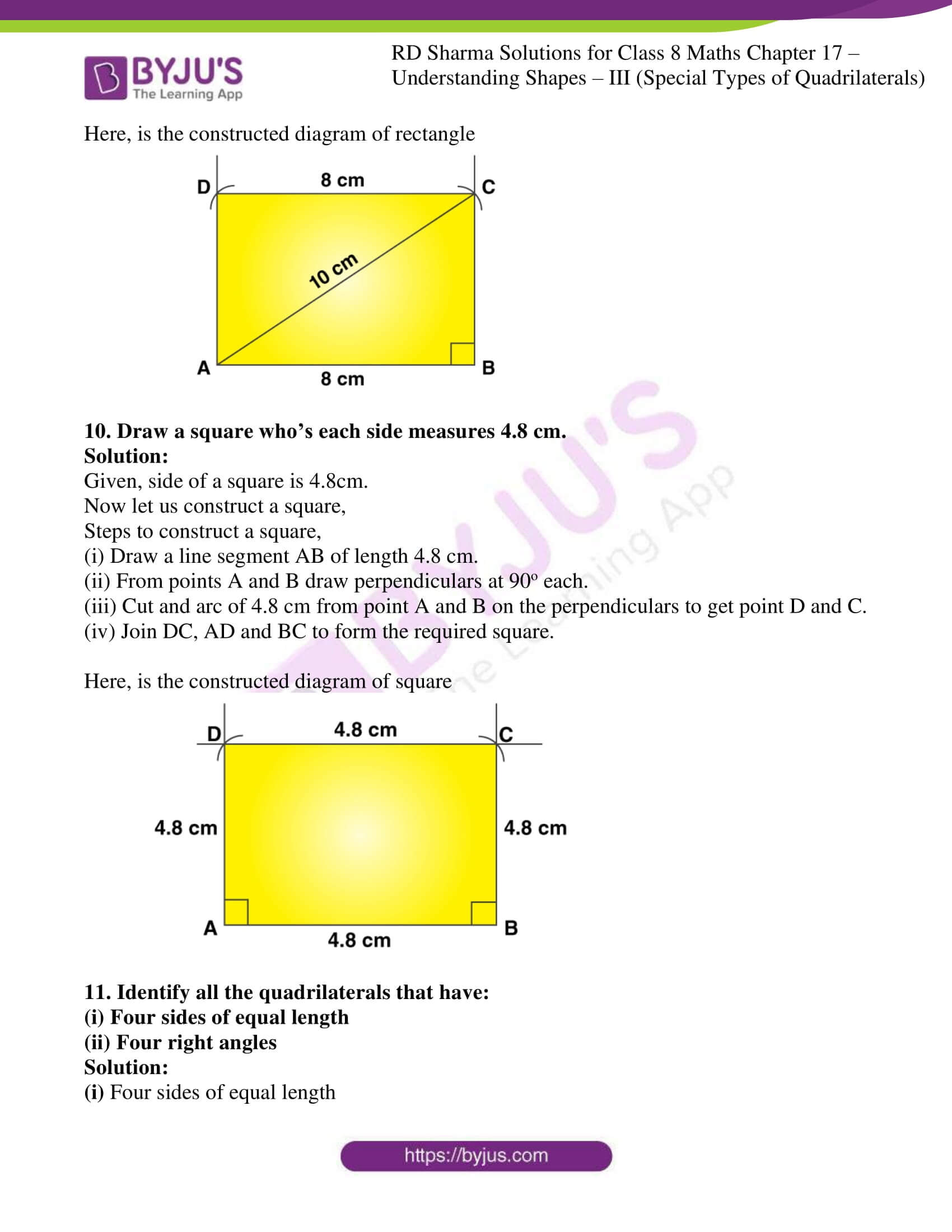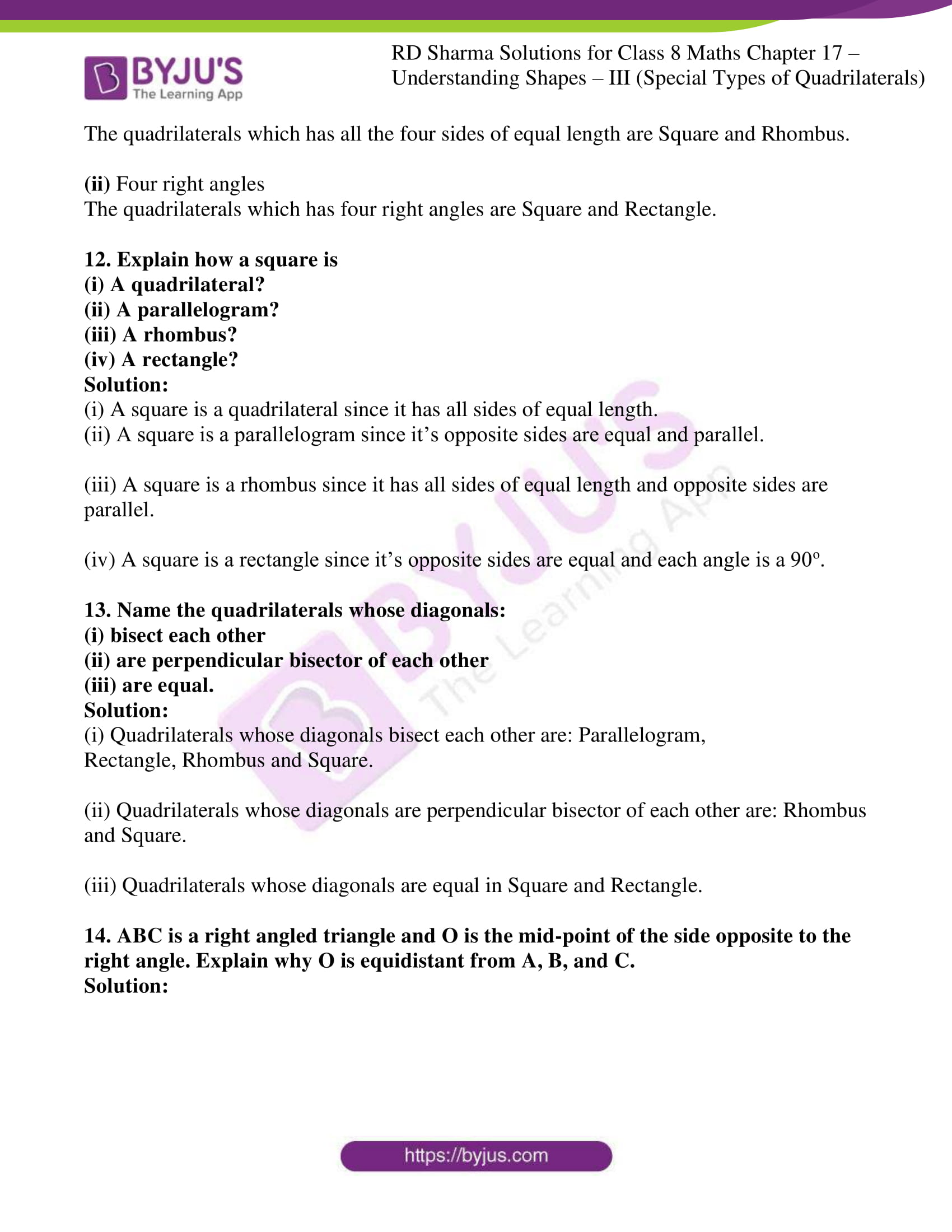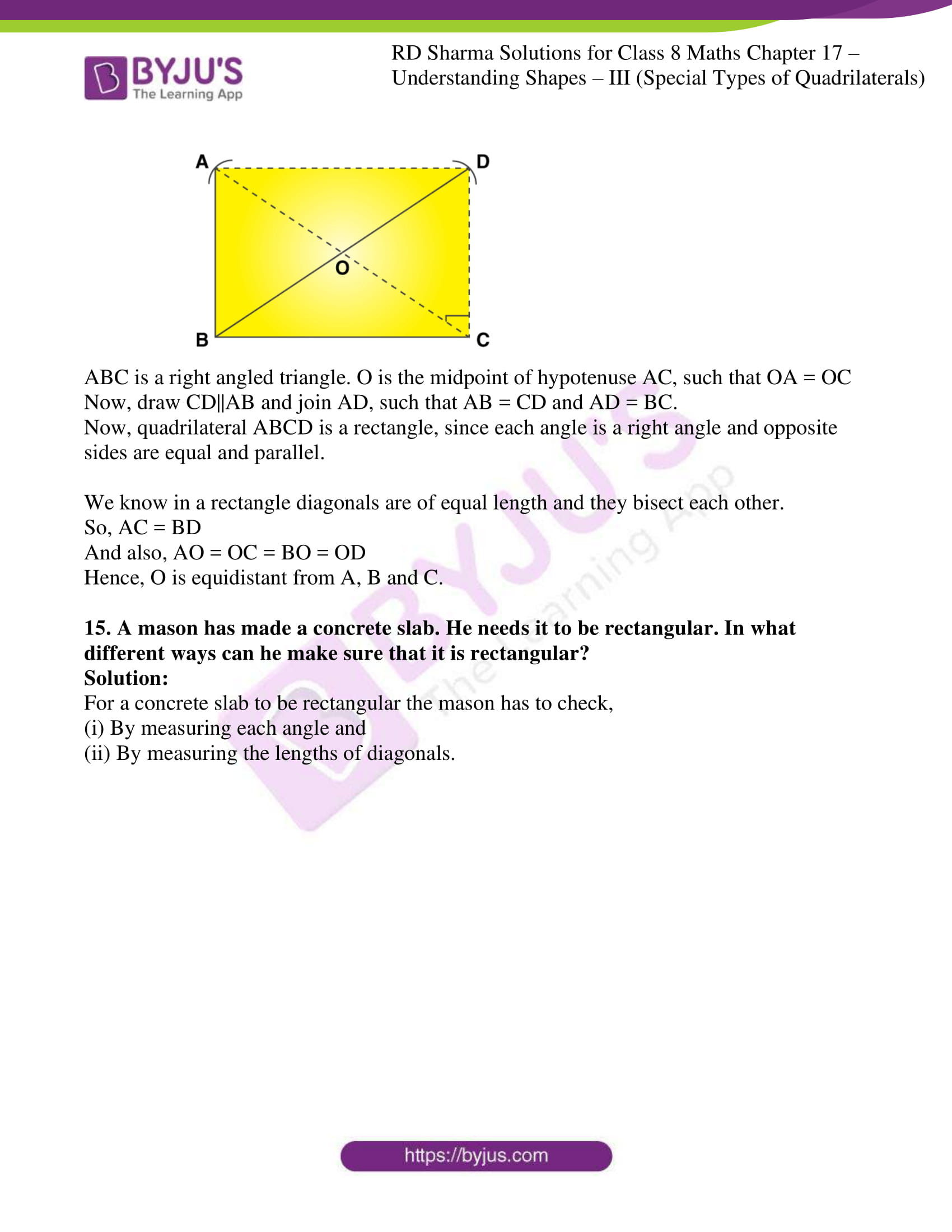### Access answers to Maths RD Sharma Solutions For Class 8 Exercise 17.3 Chapter 17 Understanding Shapes- III (Special Types of Quadrilaterals)

1. Which of the following statem
ents are true for a rectangle?
(i) It has two pairs of equal sides.
(ii) It has all its sides of equal length.
(iii) Its diagonals are equal.
(iv) Its diagonals bisect each other.
(v) Its diagonals are perpendicular.
(vi) Its diagonals are perpendicular and bisect each other.
(vii) Its diagonals are equal and bisect each other.
(viii) Its diagonals are equal and perpendicular, and bisect each other.
(ix) All rectangles are squares.
(x) All rhombuses are parallelograms.
(xi) All squares are rhombuses and also rectangles.
(xii) All squares are not parallelograms.

Solution:

(i) It has two pairs of equal sides.

True, in a rectangle two pairs of sides are equal.

(ii) It has all its sides of equal length.

False, in a rectangle only two pairs of sides are equal.

(iii) Its diagonals are equal.

True, in a rectangle diagonals are of equal length.

(iv) Its diagonals bisect each other.

True, in a rectangle diagonals bisect each other.

(v) Its diagonals are perpendicular.

False, Diagonals of a rectangle need not be perpendicular.

(vi) Its diagonals are perpendicular and bisect each other.

False, Diagonals of a rectangle need not be perpendicular. Diagonals only bisect each other.

(vii) Its diagonals are equal and bisect each other.

True, Diagonals are of equal length and bisect each other.

(viii) Its diagonals are equal and perpendicular, and bisect each other.

False, Diagonals are of equal length and bisect each other. Diagonals of a rectangle need not be perpendicular

(ix) All rectangles are squares.

False, in a square all sides are of equal length.

(x) All rhombuses are parallelograms.

True, all rhombuses are parallelograms, since opposite sides are equal and parallel.

(xi) All squares are rhombuses and also rectangles.

True, all squares are rhombuses, since all sides are equal in a square and rhombus. All squares are rectangles, since opposite sides are equal and parallel.

(xii) All squares are not parallelograms.

False, all squares are parallelograms, since opposite sides are parallel and equal.

2. Which of the following sta
tements are true for a square?
(i) It is a rectangle.
(ii) It has all its sides of equal length.
(iii) Its diagonals bisect each other at right angle.
(v) Its diagonals are equal to its sides.

Solution:

(i) It is a rectangle.

True. Since, opposite sides are equal and parallel where, each angle is right angle.

(ii) It has all its sides of equal length.

True. Since, ides of a square are of equal length.

(iii) Its diagonals bisect each other at right angle.

True. Since, diagonals of a square bisect each other at right angle.

(v) Its diagonals are equal to its sides.

False. Since, diagonals of a square are of equal length. Length of diagonals is not equal to the length of sides

3. Fill in the blanks in each of the following, so as to make the statement true :
(i) A rectangle is a parallelogram in which ________.
(ii) A square is a rhombus in which __________.
(iii) A square is a rectangle in which ___________.

Solution:

(i) A rectangle is a parallelogram in which one angle is a right angle.

(ii) A square is a rhombus in which one angle is a right angle.

(iii) A square is a rectangle in which adjacent sides are equal.

4. A window frame has one diagonal longer than the other. Is the window frame a rectangle? Why or why not?

Solution:

No, diagonals of a rectangle are equal length.

5. In a rectangle ABCD, prove that ΔACB ≅ΔCAD.

Solution:

Let us draw a rectangle,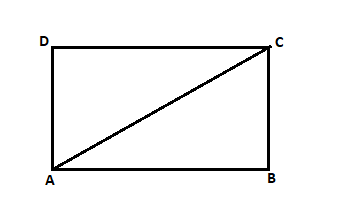In rectangle ABCD, AC is the diagonal.

AB = CD [Opposite sides of a rectangle are equal]

BC = DA

AC = CA [Common]

By using SSS congruency

6. The sides of a rectangle are in the ratio 2 : 3, and its perimeter is 20 cm. Draw the rectangle.

Solution:

In rectangle ABCD,

Given, perimeter of a rectangle = 20cm

Ratio = 2:3

So, let us consider the side as ‘x’

Length of rectangle (l) = 3x

Breadth of the rectangle (b) = 2x

We know that,

Perimeter of the rectangle = 2(length + breadth)

20 = 2(3x + 2x)

10x = 20

x = 20/10 = 2

Length of the rectangle = 3×2 = 6cm

Breadth of the rectangle = 2×2 = 4cm

Here, is the diagram of rectangle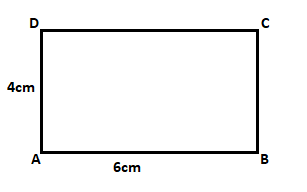7. The sides of a rectangle are in the ratio 4 : 5. Find its sides if the perimeter is 90 cm.

Solution:

In rectangle ABCD,

Given, perimeter of a rectangle = 90cm

Ratio = 4:5

So, let us consider the side as ‘x’

Length of rectangle (l) = 5x

Breadth of the rectangle (b) = 4x

We know that,

Perimeter of the rectangle = 2(length + breadth)

90 = 2(5x + 4x)

18x = 90

x = 90/18 = 5

Length of the rectangle = 5×5 = 25cm

Breadth of the rectangle = 4×5 = 20cm

Here, is the diagram of rectangle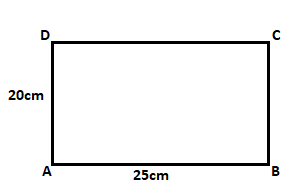8. Find the length of the diagonal of a rectangle whose sides are 12 cm and 5 cm.

Solution: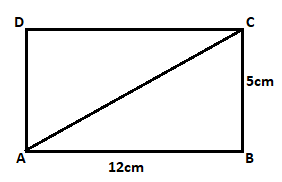In rectangle ABCD,

Given, sides of a rectangle ABCD are 5cm and 12cm

In ΔABC using Pythagoras theorem,

AC2 = AB2 + BC2

AC2 = 122 + 52

AC2 = 144 + 25

AC2 = 169

AC = √169

AC = 13cm

∴ Length of the diagonal AC is 13cm.

9. Draw a rectangle whose one side measures 8 cm and the length of each of whose diagonals is 10 cm.

Solution:

Given, one side of the rectangle is 8cm.

Length of the diagonal = 10cm

Now let us construct a rectangle,

Steps to construct a rectangle,

(i) Draw a line segment AB of length 8 cm

(ii) From point ‘A’ cut an arc of length 10 cm and mark that point as C.

(iii) From point B draw an angle of 90°, and join the arc from point A which cuts at point C.

(iv) now join AC and BC

(v) From point A draw an angle of 90° and from point C cut an arc of length 8 cm to get point D.

(vi) Join CD and AD to form required rectangle.

Here, is the constructed diagram of rectangle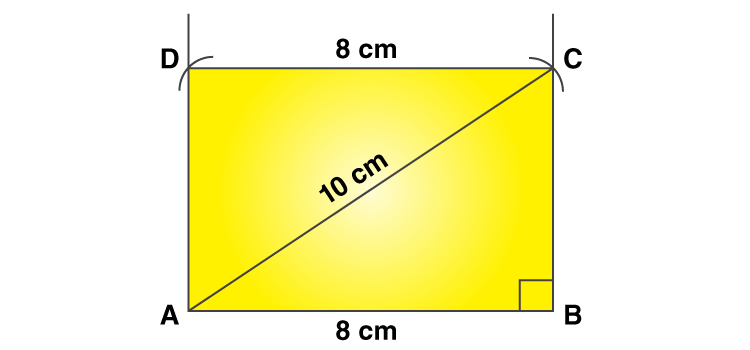10. Draw a square who’s each side measures 4.8 cm.

Solution:

Given, side of a square is 4.8cm.

Now let us construct a square,

Steps to construct a square,

(i) Draw a line segment AB of length 4.8 cm.

(ii) From points A and B draw perpendiculars at 90o each.

(iii) Cut and arc of 4.8 cm from point A and B on the perpendiculars to get point D and C.

(iv) Join DC, AD and BC to form the required square.

Here, is the constructed diagram of square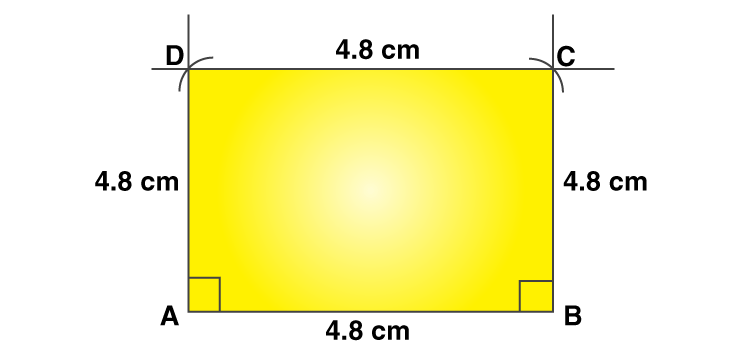11. Identify all the quadrilaterals that have:

(i) Four sides of equal length

(ii) Four right angles

Solution:

(i) Four sides of equal length

The quadrilaterals which has all the four sides of equal length are Square and Rhombus.

(ii) Four right angles

The quadrilaterals which has four right angles are Square and Rectangle.

12. Explain how a square is
(ii) A parallelogram?
(iii) A rhombus?
(iv) A rectangle?

Solution:

(i) A square is a quadrilateral since it has all sides of equal length.

(ii) A square is a parallelogram since it’s opposite sides are equal and parallel.

(iii) A square is a rhombus since it has all sides of equal length and opposite sides are parallel.

(iv) A square is a rectangle since it’s opposite sides are equal and each angle is a 90o.

13. Name the quadrilaterals whose diagonals:
(i) bisect each other
(ii) are perpendicular bisector of each other
(iii) are equal.

Solution:

(i) Quadrilaterals whose diagonals bisect each other are: Parallelogram,

Rectangle, Rhombus and Square.

(ii) Quadrilaterals whose diagonals are perpendicular bisector of each other are: Rhombus and Square.

(iii) Quadrilaterals whose diagonals are equal in Square and Rectangle.

14. ABC is a right angled triangle and O is the mid-point of the side opposite to the right angle. Explain why O is equidistant from A, B, and C.

Solution: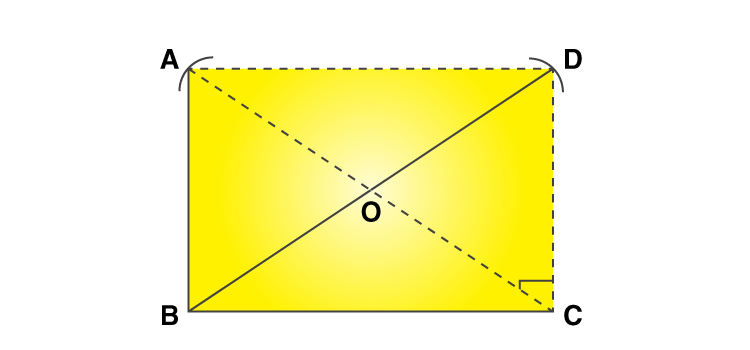ABC is a right angled triangle. O is the midpoint of hypotenuse AC, such that OA = OC

Now, draw CD||AB and join AD, such that AB = CD and AD = BC.

Now, quadrilateral ABCD is a rectangle, since each angle is a right angle and opposite sides are equal and parallel.

We know in a rectangle diagonals are of equal length and they bisect each other.

So, AC = BD

And also, AO = OC = BO = OD

Hence, O is equidistant from A, B and C.

15. A mason has made a concrete slab. He needs it to be rectangular. In what different ways can he make sure that it is rectangular?

Solution:

For a concrete slab to be rectangular the mason has to check,

(i) By measuring each angle and

(ii) By measuring the lengths of diagonals.